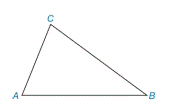Chapter 2.4, Problem 4E### Elementary Geometry for College St...

6th Edition
Daniel C. Alexander + 1 other
ISBN: 9781285195698

#### Solutions

Chapter
Section### Elementary Geometry for College St...

6th Edition
Daniel C. Alexander + 1 other
ISBN: 9781285195698
Textbook Problem
1 views

# In Exercise 1 to 4, refer to Δ A B C . On the basis of the information given, determine the measure of the remaining angle(s) of the triangle.m ∠ B = 42 ° and m ∠ A = m ∠ C

To determine

To find:

The measure of A.and C

Explanation

Given:

The given statement is,

mB=42° and mA=mC

Figure (1)

Calculation:

In a triangle, the sum of the measures of the interior angles is 180°.

The given statement is,

mB=42° and mC

The sum of the measures of the interior angles is 180°.

mA+mB+mC=180°

Substitute mC for mA and 42° for mB in the above equation.

### Still sussing out bartleby?

Check out a sample textbook solution.

See a sample solution

#### The Solution to Your Study Problems

Bartleby provides explanations to thousands of textbook problems written by our experts, many with advanced degrees!

Get Started

#### In problems 5-8, write the following sets in a second way. 8.

Mathematical Applications for the Management, Life, and Social Sciences

#### If y = f (g(x)) then y′ = f′(g′(x)).

Study Guide for Stewart's Single Variable Calculus: Early Transcendentals, 8th

#### 2 1 0 does not exist

Study Guide for Stewart's Multivariable Calculus, 8th

#### Self Check Solve: 25=3x-4.

College Algebra (MindTap Course List)